# Texas Go Math Grade 3 Lesson 8.1 Answer Key Multiply with 7

Refer to our Texas Go Math Grade 3 Answer Key Pdf to score good marks in the exams. Test yourself by practicing the problems from Texas Go Math Grade 3 Lesson 8.1 Answer Key Multiply with 7.

## Texas Go Math Grade 3 Lesson 8.1 Answer Key Multiply with 7

Essential Question
What strategies can you use to multiply with 7?

Unlock the Problem
Logan’s family has a new puppy. Logan takes a turn walking the puppy once a day. How many times will Logan walk the puppy in 4 weeks?
Find 4 × 7.

• How often does Logan walk the puppy?
Logan takes a turn walking the puppy once a day.

Explanation:
Logan takes a turn walking the puppy once a day.

• How many days are in 1 week?
There are 7 days a week.

Explanation:
Number of days in a week = 7.

One Way
Use the Commutative Property of Multiplication.
If you know 7 × 4, you can use that fact to find 4 × 7.
You can change the order of the factors and the product is the same.
7 × 4 = ___, so, 4 × 7 = ___.
so, Logan will walk the puppy __ times in 4 weeks.

Math Talk
Mathematical Processes
Explain two other ways you can break apart the 4 × 7 array.
One way:
4 × 7 = (2 × 7) + ( 2× 7)  = 28.
Other way:
(4 × 3) + (4 × 4) = 28.

Explanation:
One way:
4 × 7 = (2 × 7) + ( 2× 7) = 14 + 14 = 28.
Other way:
(4 × 3) + (4 × 4) = 12 + 16 = 28.

Other Ways
A. Use the Distributive Property.
Step 1
Complete the array to show 4 rows of 7.Step 2
Draw a line to break the array into two smaller arrays for facts you know.

Step 3
Multiply the facts for the smaller arrays. Add the products.
So, 4 × 7 = ___.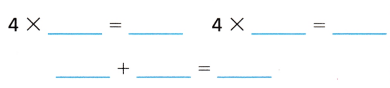B. Use a fact you know.
Multiply. 4 × 7 = ___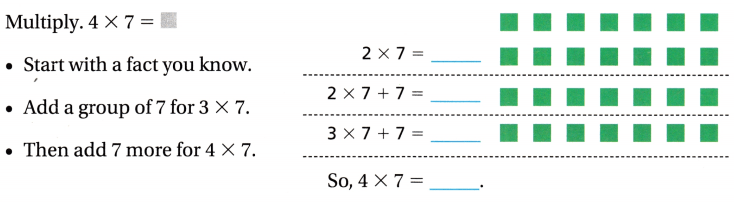Share and Show

Question 1.
Explain how you could break apart an array to find 6 × 7. Draw an array to show your work.
6 × 7 = (3 × 7) + (2 × 7) + (1 × 7) = 42.

Explanation:
6 × 7 = (3 × 7) + (2 × 7) + (1 × 7)
= 21 + 14 + 7
= 35 + 7
= 42.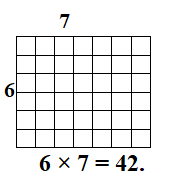Math Talk
Mathematical Processes
How can you use doubles to find 8 × 7 ?
Doubles to find 8 × 7:
(2 × 7) + (2 × 7) + (2 × 7) + (2 × 7)
= 14 + 14 + 14 + 14
= 28 + 14 + 14
= 42 + 14
= 56.

Explanation:
Doubles to find 8 × 7:
2 × 7 = 14.

Find the product.

Question 2.
9 × 7 = ___
9 × 7 = 63.

Explanation:
9 × 7 = 63.

___ = 5 × 7
35 = 5 × 7.

Explanation:

Question 4.
___ = 7 × 3
21= 7 × 3.

Explanation:
7 × 3 = 21.

Question 5.
8 × 7 = ___
8 × 7 = 56.

Explanation:
8 × 7 = 56.

Question 6.
___ = 7 × 7
49 = 7 × 7

Explanation:
7 × 7 = 49.

Question 7.
6 × 7 = ___
6 × 7 = 42.

Explanation:
6 × 7 = 42.

Question 8.
___ = 7 × 10
70 = 7 × 10

Explanation:
7 × 10 = 70.

Question 9.
___ = 7 × 2
14 = 7 × 2

Explanation:
7 × 2 = 14.

Question 10.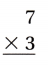Explanation:
7 × 3 = 21.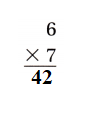Explanation:
6 × 7 = 42.

Question 12.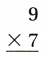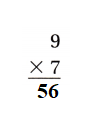Explanation:
9 × 7 = 63.

Question 13.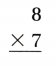Explanation:
8 × 7 = 56.

Question 14.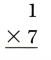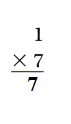Explanation:
1 × 7 = 7.

Question 15.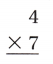Explanation:
4 × 7 = 28.

Question 16.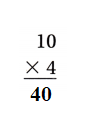Explanation:
10 × 4 = 40.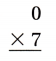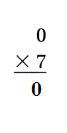Explanation:
0 × 7 = 0.

Question 18.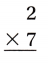Explanation:
2 × 7 = 14.

Question 19.Explanation:
5 × 7 = 35.

Question 20.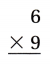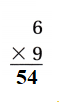Explanation:
6 × 9 = 54.

Question 21.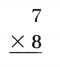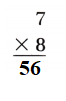Explanation:
7 × 8 = 56.

Problem Solving
Use the table for 22-24.
Question 22.
Olivia has a dog named Rusty. How many baths will Rusty have in 7 months?Number of times Rusty takes bath in a 7 months = 14.

Explanation:
Number of times Rusty takes bath in a month = 2.
Number of times Rusty takes bath in a 7 months = 7 × Number of times Rusty takes bath in a month
= 7 × 2
= 14 times.

H.O.T. Multi-Step How many more cups of water than food will Rusty get in 1 week?7 more cups of water than food will Rusty get in 1 week.

Explanation:
Number of cups of water Rusty takes in a day = 4.
Conversion: 1 Week = 7 days.
=> Number of cups of water Rusty takes in a week = 7 × Number of cups of water Rusty takes in a day
= 7 × 4
= 28.
Number of cups of food Rusty takes in a day = 3.
=> Number of cups of food Rusty takes in a week = 7 × Number of cups of food Rusty takes in a day
= 7 × 3
= 21.
Difference:
Number of cups of water Rusty takes in a week – Number of cups of food Rusty takes in a week
= 28 – 21
= 7.

Question 24.
H.O.T. Write Math Jacob’s dog Midnight eats 28 cups of food in a week. Midnight eats the same amount each day. In one day, how many more cups of food will Midnight eat than Rusty? Explain.
25 more cups of food will Midnight eat than Rusty.

Explanation:
Number of cups of food Jacob’s dog Midnight eats = 28.
Number of cups of food Rusty takes in a day = 3.
Difference:
Number of cups of food Jacob’s dog Midnight eats – Number of cups of food Rusty takes in a day
= 28 – 3
= 25.

Question 25.
Jose walks his dog 10 miles every week. How many miles do they walk in 7 weeks?
Number of miles Jose walks his dog in 7 weeks = 70.

Explanation:
Number of miles every week Jose walks his dog = 10.
Conversion:
1 Week = 7 days.
=> Number of miles Jose walks his dog in 7 weeks = 7 × Number of miles every week Jose walks his dog
= 7 × 10
= 70.

Question 26.
Multi-Step Apply Ethan takes his dog Zoey for a 3-mile walk twice a day. How many miles do they walk in one week?
Number of miles they walk in one week = 42.

Explanation:
Number of miles Zoey walks a day = 3.
Number of times Zoey walks a day = 2.
Total number of miles Zoey walks a day = Number of miles Zoey walks a day × Number of times Zoey walks a day
= 3 × 2
= 6.
Conversion:
1 Week = 7 days.
Number of miles they walk in one week = 7 × Total number of miles Zoey walks a day
= 7 × 6
= 42.

Fill in the bubble for the correct answer choice.
Question 27.
Sebastian the Sailor sails the Seven Seas. He drops 5 gold coins into each sea for good luck. How many gold coins does Sebastian the Sailor drop into the seas?
(A) 45
(B) 35
(C) 25
(D) 75
Number of gold coins Sebastian the Sailor drops into the seas = 35.
(B) 35.

Explanation:
Number of seas Sebastian the Sailor sails = 7.
Number of gold coins into each sea for good luck = 5.
Number of gold coins Sebastian the Sailor drops into the seas = Number of seas Sebastian the Sailor sails × Number of gold coins into each sea for good luck
= 7 × 5
= 35.

Question 28.
Emilia makes 7 huge sandwiches for the class picnic. She uses 3 slices of bread in each sandwich. How many slices of bread does Emilia use for the sandwiches?
(A) 10
(B) 18
(C) 21
(D) 14
Number of slices of bread Emilia uses for the sandwiches = 21.
(C) 21.

Explanation:
Number of huge sandwiches for the class picnic Emilia makes = 7.
Number of slices of bread in each sandwich = 3.
Number of slices of bread Emilia uses for the sandwiches = Number of huge sandwiches for the class picnic Emilia makes × Number of slices of bread in each sandwich
= 7 × 3
= 21.

Question 29.
Multi-Step Anders makes 7 fruit cups. He puts 2 green grapes, 2 red grapes, and 2 black grapes in each fruit cup. How many grapes does Anders use for the fruit cups?
(A) 42
(B) 28
(C) 13
(D) 14
Number of grapes Anders uses for the fruit cups = 42.
(A) 42

Explanation:
Number of fruit cups Anders makes = 7.
Number of green grapes he puts = 2.
Number of red grapes he puts = 2.
Number of black grapes he puts = 2.
Number of grapes Anders uses for the fruit cups = Number of fruit cups Anders makes(Number of green grapes he puts + Number of red grapes he puts + Number of black grapes he puts)
= 7(2 + 2 + 2)
= 7(4 + 2)
= 7 × 6
= 42.

Texas Test Prep

Question 30.
Madison walks her dog 4 miles a day. How many miles does she walk in one week?
(A) 5 miles
(B) 32 miles
(C) 28 miles
(D) 11 miles
Number of miles does she walk in one week = 28.
(C) 28 miles.

Explanation:
Number of miles Madison walks her dog a day = 4.
Conversion:
1 week = 7 days.
Number of miles does she walk in one week = 7 × Number of miles Madison walks her dog a day
= 7 × 4
= 28.

### Texas Go Math Grade 3 Lesson 8.1 Homework and Practice Answer Key

Find the product.

Question 1.
6 × 7 = ___
6 × 7 = 42.

Explanation:
6 × 7 = 42.

Question 2.
10 × 7 = ___
10 × 7 = 70.

Explanation:
10 × 7 = 70.

Question 3.
7 × 3 = ___
7 × 3 = 21.

Explanation:
7 × 3 = 21.

Question 4.
7 × 9 = ___
7 × 9 = 63.

Explanation:
7 × 9 = 63.

2 × 7 = ___
2 × 7 = 14.

Explanation:-
2 × 7 = 14.

Question 6.
1 × 7 = ___
1 × 7 = 7.

Explanation:
1 × 7 = 7.

Question 7.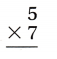Explanation:
5 × 7 = 35.

Question 8.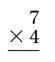Explanation:
7 × 4 = 28.

Question 9.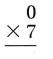Explanation:
0 × 7 = 0.

Question 10.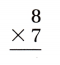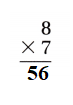Explanation:
8 × 7 = 56.

Question 11.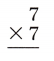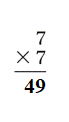Explanation:
7 × 7 = 49.Explanation:
7 × 6 = 42.

Use the table for 13-15.

Question 13.
How many pails of food do the elephants eat in one week?Number of pails of food elephant eats in a week = 63.

Explanation:
Number of pails of food elephant eats in a day = 9.
Number of days in a week = 7.
Number of pails of food elephant eats in a week = Number of days in a week  × Number of pails of food elephant eats in a day
= 7 × 9
= 63.

Question 14.
If the zoo keepers increased the lion’s feed by 2 pails, how many pails of food would the lions eat in one week?
Number of pails of food would lion eats in a week = 56.

Explanation:
Number of pails of food lion eats in a day = 4.
Number of days in a week = 7.
Number of pails of food lion eats in a week = Number of days in a week  × Number of pails of food lion eats in a day
= 7 × 4
= 28.
If the zoo keepers increased the lion’s feed by 2 pails.
=> Number of pails of food would lion eats in a week = 2 × Number of pails of food lion eats in a week
= 2 × 28
= 56.

Question 15.
How many more pails of food do the zebras eat in one week than the lions?
21 more pails of food do the zebras eat in one week than the lions.

Explanation:
Number of pails of food lion eats in a week = 28.
Number of pails of food zebra eats in a day = 7.
Number of days in a week = 7.
Number of pails of food zebra eats in a week = Number of days in a week  × Number of pails of food zebra eats in a day
= 7 × 7
= 49.
Difference:
Number of pails of food zebra eats in a week – Number of pails of food lion eats in a week
= 49 – 28
= 21.

Problem Solving
Question 16.
Mark works 3 hours each day feeding the animals and 4 hours in the zoo store. How many hours does Mark work in 5 days? Explain.
Number of hours Mark work in 5 days = 35.

Explanation:
Number of hours each day feeding the animals Mark works = 3.
Number of hours each day in the zoo store Mark works = 4.
Number of days = 5.
Number of hours Mark work in 5 days = Number of days (Number of hours each day feeding the animals Mark works + Number of hours each day in the zoo store Mark works)
= 5 (3 + 4)
= 5 × 7
= 35.

Texas Test Prep
Lesson Check
Question 17.
Sudi serves pancakes at the town picnic. He puts 3 pancakes on each of 7 plates. How many pancakes does Sudi serve?
(A) 10
(B) 21
(C) 28
(D) 20
Number of pancakes Sudi serve = 21.
(B) 21.

Explanation:
Number of pan cakes he puts on each plate = 3.
Number of plates = 7.
Number of pancakes Sudi serve = Number of pan cakes he puts on each plate × Number of plates
= 3 × 7
= 21.

Question 18.
Rosa buys 3 books and 4 magazines. Each one costs $4. How much does Rosa spend on books and magazines? (A)$28
(B) $11 (C)$12
(D) $24 Answer: Amount of money Rosa spend on books and magazines =$28.
(A) $28. Explanation: Number of books Rosa buys = 3. Number of magazines Rosa buys = 4. Cost of each =$4.
Amount of money Rosa spend on books and magazines = Cost of each  ( Number of books Rosa buys + Number of magazines Rosa buys)
= $4 (3 + 4) =$4 × 7
= \$28.

Question 19.
Non uses 9 yards of yarn to make a cat toy. 1f she makes 7 toys, how many yards of yarn does Non need?
(A) 72 yards
(B) 16 yards
(C) 63 yards
(D) 56 yards
Number of yards of yarn Non need = 63.
(C) 63 yards.

Explanation:
The number of yards of yarn to make a cat toy Non uses = 9.
Number of toys she makes = 7.
Number of yards of yarn Nonneed = Number of yards of yarn to make a cat toy Nonuses × Number of toys she makes
= 9 × 7
= 63.

Go Math Practice and Homework Lesson 8.1 Answer Key Question 20.
One trip on a sky ride at the zoo takes 8 minutes. How many minutes does the ride take for 7 trips?
(A) 42 minutes
(B) 49 minutes
(C) 15 minutes
(D) 56 minutes
Number of minutes the ride takes for 7 trips = 56.
(D) 56 minutes.

Explanation:
Number of minutes one trip on a sky ride at the zoo takes = 8.
Number of trips = 7.
Number of minutes the ride take for 7 trips = Number of trips  × Number of minutes one trip on a sky ride at the zoo takes
= 7 × 8
= 56.

Question 21.
Multi-Step An album holds 6 photos on one page. A second album holds 4 photos on a page. If Nick fills 7 pages in each album, how many photos does Nick have in all?
(A) 28
(B) 70
(C) 42
(D) 17
Number of photos Nick have in all = 70.
(B) 70.

Explanation:
Number of photos on one page an album holds = 6.
Number of photos on one page an second album holds = 4.
If Nick fills 7 pages in each album.
=> Number of photos Nick have in all = 7 (Number of photos on one page an album holds + Number of photos on one page an second album holds)
= 7(6 + 4)
= 7 × 10
= 70.

Multi-Step Alexander makes a tile design with 3 red tiles, 1 yellow tile and 3 purple tiles. If he makes 5 designs, how many tiles does Alexander need in all?
(A) 30
(B) 18
(C) 35
(D) 12
Number of tiles does Alexander need in all = 35.
(C) 35.

Explanation:
Number of red tiles Alexander makes a tile design with = 3.
Number of yellow tiles Alexander makes a tile design with = 1.
Number of purple tiles Alexander makes a tile design with = 3.
Number of designs he makes = 5.
Number of tiles does Alexander need in all = Number of designs he makes (Number of red tiles Alexander makes a tile design with + Number of yellow tiles Alexander makes a tile design with + Number of purple tiles Alexander makes a tile design with)
= 5 (3 + 1 + 3)
= 5 (4 + 3)
= 5 × 7
= 35.

Scroll to Top